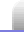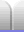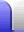# NCEA Level 2, 2019 Mathematics exam

Some of the questions from the exam held on Thursday 21 November 2019, as posted in this NZ Herald article.

### Algebra

Unknown question number.

Basically, a rectangle is divided into two areas. The shaded rectangle has an area of 9 cm2. A diagram is given with some dimensions. Find the area of the larger rectangle.Radio NZ posted a couple of videos here and here with University of Auckland's Padraic Bartlett explaining the problem. Sadly the maths professor has very messy writing.

y(y – 8) = 9
y2 – 8y – 9 = 0
(y – 9)(y + 1) = 0
y = 9 or y = -1

It's a rectangle, so y must be positive, hence y = 9. The shaded rectangle is thus 1 cm wide and 9 cm tall.

x = y – 8 + y + 2
x = 2y – 6
x = 2(9) – 6
x = 18 – 6
x = 12

This is the width of the rectangle. The height of the rectangle is x – 4 = 8. Hence the larger rectangle is 12 cm wide and 8 cm tall. But the height of the shaded rectangle is 9 cm. That means the distance from the bottom of the shaded rectangle to the bottom of the larger rectangle is -1 cm!

Comment:

It's incredible that mistakes are still making it through the NCEA exam preparation process. Hasn't NZQA learned anything!? It's been making mistakes for years!

As has been pointed out elsewhere, the area of the rectangle can be found even with the mistake, but the mistake is a trap for more capable students, since they are more likely to check their answer and find it cannot possibly be right, which could lead to them wasting a lot of time trying to figuer out where they went wrong. It's a maths exam, not a psychology experiment!

I don't see any problem with the way the rectangle is labelled. Students should be aware they need to be careful in what they are doing, and what they are using. The marking scheme should allow for the relative importance of using the wrong dimension.

### Algebra

QUESTION ONE (c)If p(x) is a perfect square then it will only have one repeated root, which means the discriminant equals zero. This allows us to make an equation which can be solved.

b2 - 4ac = 0
(m + 1)2 – 4(2m – 1)(m – 4) = 0
(m2 + 2m + 1) – 4(2m2 – 9m + 4) = 0
m2 + 2m + 1 – 8m2 + 36m – 16 = 0
-7m2 + 38m – 15 = 0

Now use the quadratic formula or hope the answer is a whole number and try to factorise.

-38 ± √[382 – 4(-7)(-15)]
m = ——————————————
-14

-38 ± √
m = —————————
-14

-38 ± 32
m = —————
-14

-6
m = —— = 3/7
-14

-70
or m = —— = 5
-14

Factorising will be easier with a positive x2 term.

7m2 – 38m + 15 = 0

We're looking for two negative numbers which multiply to 15 and (after one is multiplied by 7) have a sum of -38. (We don't need to continue checking the options after we find the sum we're looking for.)

1x 7x Sum
1 15 106
3 5 38
5 3 26
15 1 22

(7m – 3)(m – 5) = 0

7m – 3 = 0
m = 3/7

or m – 5 = 0
m = 5

But wait. We have two solutions, and we were told it's a perfect square. Are both solutions perfect squares? To find out we'll have to test them both.

(2m – 1)x2 + (m + 1)x + (m – 4)

(2(3/7) – 1)x2 + ((3/7) + 1)x + ((3/7) – 4)
-1/7x2 + 10/7x25/7
-1/7(x2 + 10x – 25)
-1/7(x – 5)2
Not a perfect square!

(2(5) – 1)x2 + ((5) + 1)x + ((5) – 4)
9x2 + 6x + 1
(3x + 1)2
Perfect square.

We therefore reject 3/7 as a solution and conclude that m = 5 (only).

Comment:

This final conclusion probably isn't required for full marks whatever it is NCEA actually uses (but with NCEA exams being famed for inconsistencies and errors, you never know). Rejecting one solution after all the rest of the working adds up to a lot of work for a single NCEA 2 sub-question, but really is incomplete without it.

However, we can only reject 3/7 as a solution because complex numbers are not part of the NCEA Level 2 syllabus. It actually is a solution if we include √ ( -1/7 ) inside the perfect square instead of just being a coefficient outside it. I regard this as an error.

Quite simply, NZQA was very careless in having a Level 2 answer involving complex numbers. It would have been very easy to give an indication of whether that solution should be included. For example, the question could have just stated "m is an integer".

### Algebra

QUESTION TWO (e)First factorise with a placeholder, a. We'll get rid of it later.

(x + a)(x + an) = 0
x2 + anx + ax + a2n = 0
x2 + a(n + 1)x + a2n = 0

 p = a(n + 1) a = p / (n + 1) q = a2n a2 = q / n

There are (at least) a couple of approaches from here.

 Make an equation by letting these two equal each other to remove a, then rearrange. p2 / (n + 1)2 = q / n p2n = q(n + 1)2 p2n = q(n2 + 2n + 1) p2n = qn2 + 2qn + q qn2 + 2qn – p2n + q = 0 qn2 + (2q – p2)n + q = 0 That's quite efficient, but it's not necessarily obvious why making the equation is a good idea. Substitute the two roots into x in the original equation, then replace a and a2 with their equal expressions. x2 + px + q = 0 (-a)2 + p(-a) + q = 0 a2 + -ap + q = 0 q / n + -[p / (n + 1)]p + q = 0 q / n – p2 / (n + 1) + q = 0 (-an)2 + p(-an) + q = 0 a2n2 + -apn + q = 0 (q / n)n2 + -[p / (n + 1)]pn + q = 0 qn – p2n / (n + 1) + q = 0 Start rearranging. The first result can be changed into the second simply by multiplying by n. q / n – p2 / (n + 1) + q = 0 q – p2n / (n + 1) + qn = 0 q(n + 1) – p2n + qn(n + 1) = 0 qn + q – p2n + qn2 + qn = 0 qn2 + 2qn – p2n + q = 0 qn2 + (2q – p2)n + q = 0

Comment:

I found this one quite tricky and really had to think to figure out how to get an equation that could be manipulated into the required form. The maths itself is pretty straight-forward. The question will likely show which students really know how to apply what they know.

### Calculus

QUESTION ONE (b)Parallel lines have the same gradient, so rearrange linear equation to find gradient of the tangent (using y = mx + c) and differentiate the function to give its gradient function. Equate the two gradients to get an equation to solve.

y = 8x – 6

y' = 12x2 – 4

12x2 – 4 = 8
12x2 = 12
x2 = 1
x = ±1

Substitute these x values into original function to get y values.

Coordinates of two points are (-1, 4) and (1, 4)

Comment:

This was a straight-forward question.

### Calculus

QUESTION ONE (c)dV / dr = 4πr2 = 25π
4r2 = 25
r2 = 25 / 4
r = 5/2

A radius of -5/2 is not possible, so we don't take the negative root. Don't forget to state the solution clearly.

Comment:

This is a very simple question, practically a freebie. I got the solution and wondered if that was really all that was required.

### Calculus

QUESTION THREE (c)The gradient is rise/run, which for a line from the origin to any particular point are just the coordinates of the point. But we can also get an expression for the gradient by differentiating f(x); this is calculus, after all.

f'(x) = 3x2 – 6x – 4

y / x = f(x) / x
= (x3 – 3x2 – 4x) / x
= x2 – 3x – 4

f'(x) = y / x
3x2 – 6x – 4 = x2 – 3x – 4
2x2 – 3x = 0
x(2x – 3) = 0
x = 0 or 2x – 3 = 0
x = 0 or x = 3/2

Substituting these values into f(x) gives the points.

A(0, 0) and B(1.5, -9.375)

The equations of the tangents are straight forward: y = mx + c, where c = 0 since the tangent goes through the origin.

The gradient of the first tangent will need the use of f'(x).

y = f'(x)x
y = -4x

Trying to use the coordinates for that gradient – rise over run – gives 0/0, which is undefined. It serves as a reminder that messing with infinity gives weird results.

The gradient of the second tangent can be found from the coordinates.

y = -9.375/1.5 x
y = -6.25x

Comment:

This one was straight-forward after figuring out how to make an equation using the coordinates of the point. It shouldn't take much figuring out, but again, this question may test which students understand the content well enough to be able to apply it.

It may not be immediately obvious why a cubic graph that passes through the origin has another tangent which also passes through the origin; y = x3 doesn't, for example. It's because the point of inflexion between the two local extrema is not itself at the origin. If the two non-origin roots (the x-intercepts of the cubic) were equally spaced either side of the origin there would not be a second tangent which passes through the origin.Home Astronomy Chemistry Electronics & Computers Mathematics Physics Field Trips Turn on javascript for email link